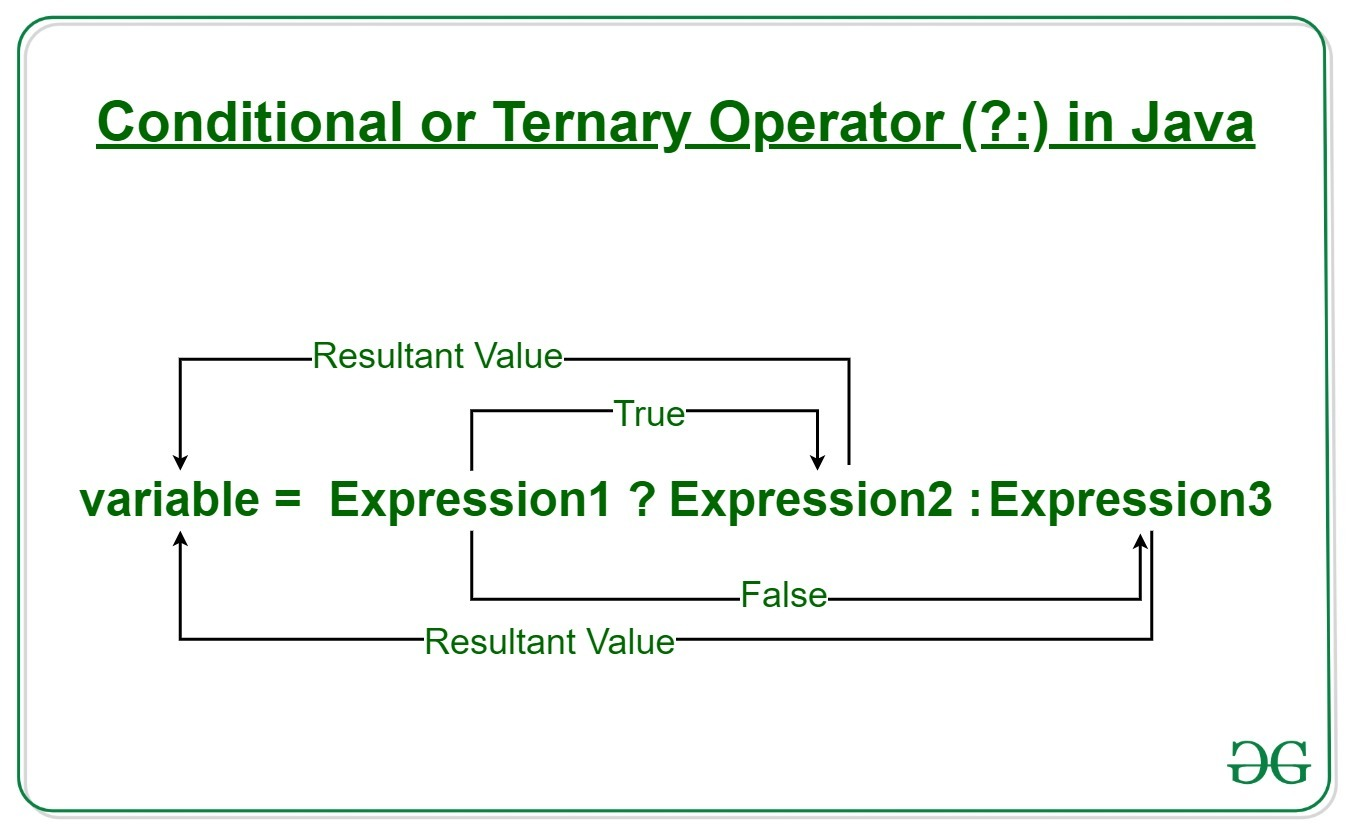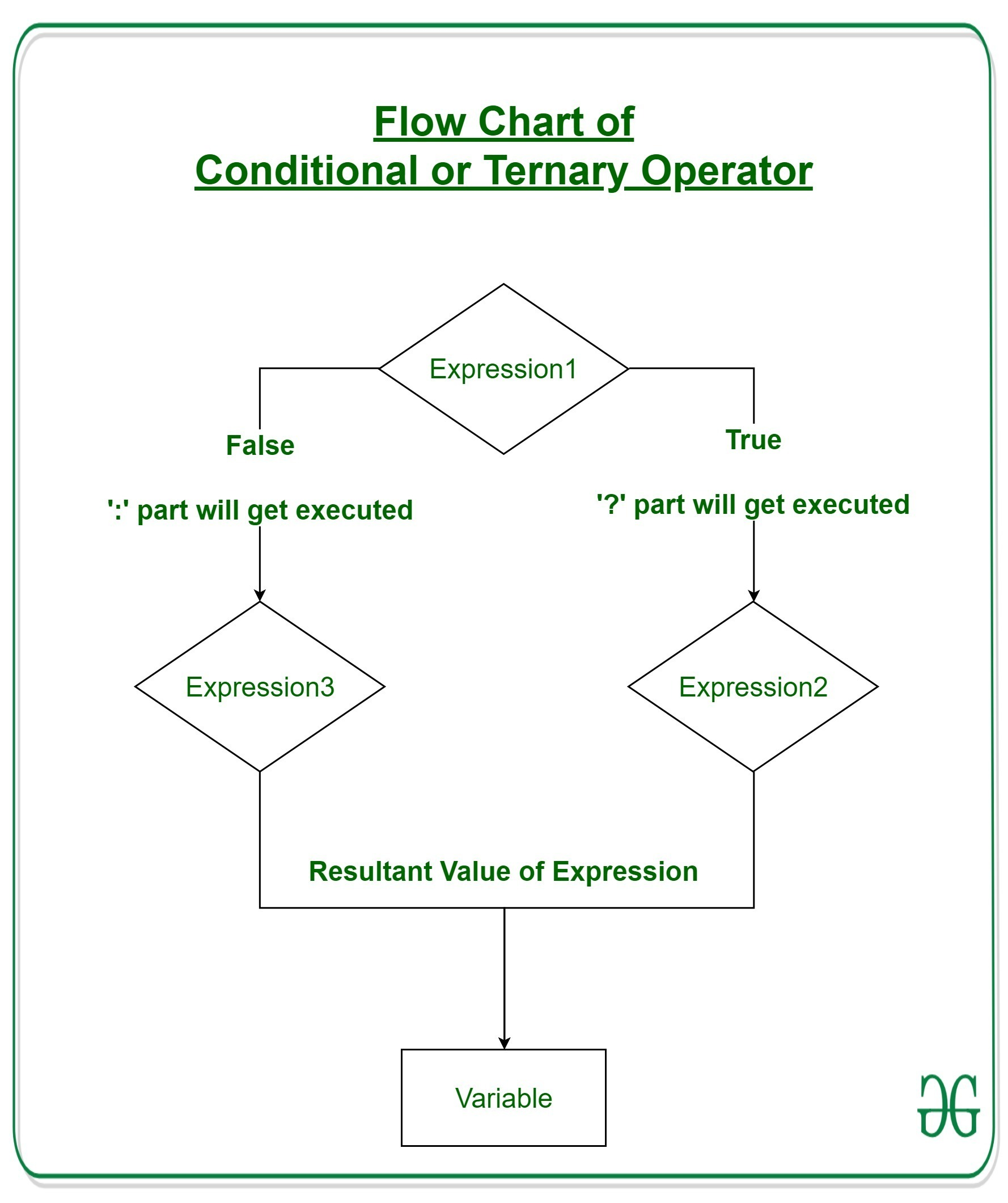Related Articles

# Java Ternary Operator with Examples

• Difficulty Level : Basic
• Last Updated : 13 Jul, 2021

Operators constitute the basic building block to any programming language. Java too provides many types of operators which can be used according to the need to perform various calculation and functions be it logical, arithmetic, relational etc. They are classified based on the functionality they provide. Here are a few types:

1. Arithmetic Operators
2. Unary Operators
3. Assignment Operator
4. Relational Operators
5. Logical Operators
6. Ternary Operator
7. Bitwise Operators
8. Shift Operators

This article explains all that one needs to know regarding the Ternary Operator.

Ternary Operator

Java ternary operator is the only conditional operator that takes three operands. It’s a one-liner replacement for if-then-else statement and used a lot in Java programming. We can use the ternary operator in place of if-else conditions or even switch conditions using nested ternary operators. Although it follows the same algorithm as of if-else statement, the conditional operator takes less space and helps to write the if-else statements in the shortest way possible.Syntax:

`variable = Expression1 ? Expression2: Expression3`

If operates similarly to that of the if-else statement as in Exression2 is executed if Expression1 is true else Expression3 is executed.

```if(Expression1)
{
variable = Expression2;
}
else
{
variable = Expression3;
}```

Example:

```num1 = 10;
num2 = 20;

res=(num1>num2) ? (num1+num2):(num1-num2)

Since num1<num2,
the second operation is performed
res = num1-num2 = -10```

Flowchart of Ternary OperationExample 1:

## Java

 `// Java program to find largest among two``// numbers using ternary operator` `import` `java.io.*;` `class` `Ternary {``    ``public` `static` `void` `main(String[] args)``    ``{` `        ``// variable declaration``        ``int` `n1 = ``5``, n2 = ``10``, max;` `        ``System.out.println(``"First num: "` `+ n1);``        ``System.out.println(``"Second num: "` `+ n2);` `        ``// Largest among n1 and n2``        ``max = (n1 > n2) ? n1 : n2;` `        ``// Print the largest number``        ``System.out.println(``"Maximum is = "` `+ max);``    ``}``}`
Output:
```First num: 5
Second num: 10
Maximum is = 10```

Example 2:

## Java

 `// Java code to illustrate ternary operator` `import` `java.io.*;` `class` `Ternary {``    ``public` `static` `void` `main(String[] args)``    ``{` `        ``// variable declaration``        ``int` `n1 = ``5``, n2 = ``10``, res;` `        ``System.out.println(``"First num: "` `+ n1);``        ``System.out.println(``"Second num: "` `+ n2);` `        ``// Performing ternary operation``        ``res = (n1 > n2) ? (n1 + n2) : (n1 - n2);` `        ``// Print the largest number``        ``System.out.println(``"Result = "` `+ res);``    ``}``}`
Output:
```First num: 5
Second num: 10
Result = -5```

Attention reader! Don’t stop learning now. Get hold of all the important Java Foundation and Collections concepts with the Fundamentals of Java and Java Collections Course at a student-friendly price and become industry ready. To complete your preparation from learning a language to DS Algo and many more,  please refer Complete Interview Preparation Course.

My Personal Notes arrow_drop_up